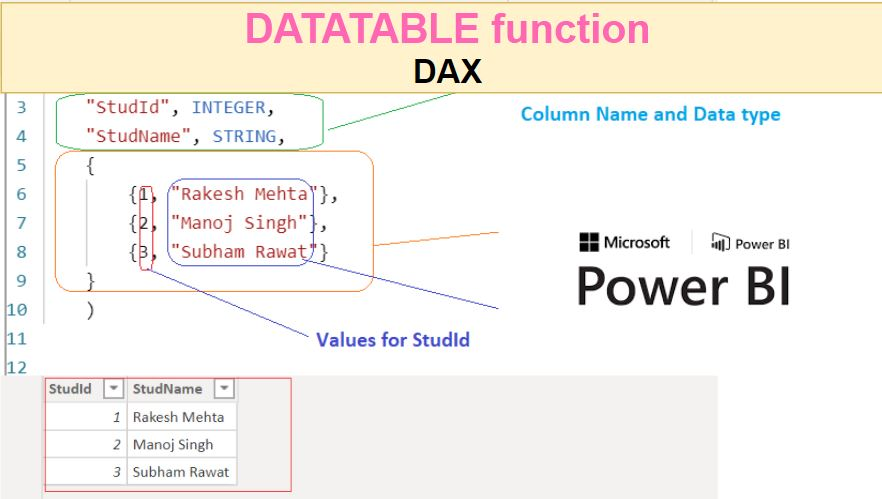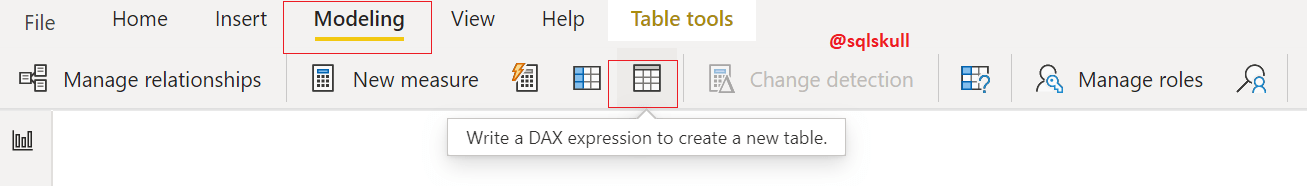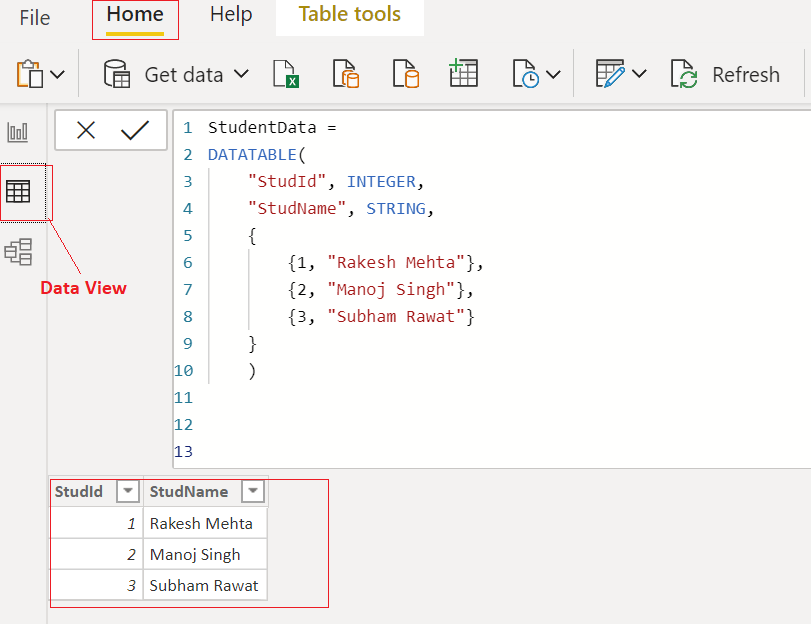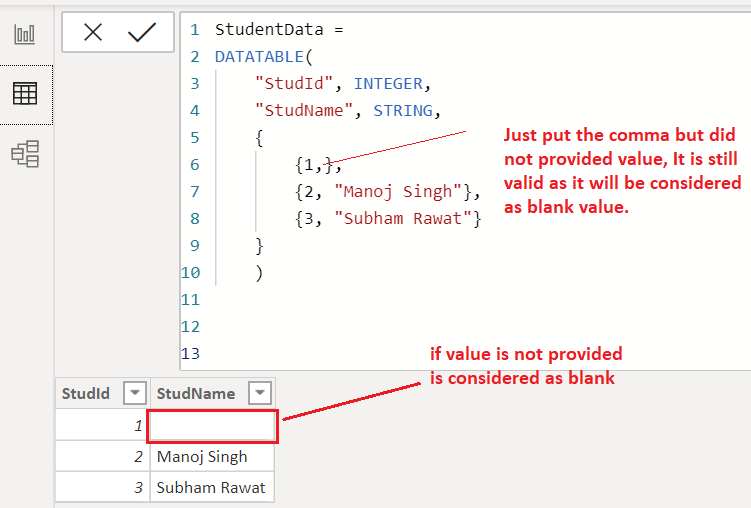Home » DATATABLE Function DAX

# DATATABLE Function DAX### DATATABLE function is a Power BI Table manipulation function in DAX which allows us to create a table.

Using Datatable function, You can define column and datatype for table and add values to them.

```SYNTAX

DATATABLE

(column_Nname1, datatype1,

column_name2, datatype2...,

{{value1, value2...}, {valueN, valueN+1 ..}...}

)```

column_name is a Dax expression that returns a table.

datatype is a datatype of column it could be an INTEGER, DOUBLE, STRING, BOOLEAN, CURRENCY, DATETIME.

value is data values that will be in the table, missing value will be considered as BLANK().

Lets look at an example of using DATATABLE Function.

Creating a DATATABLE, go to modeling tab in ribbon bar then click on DAX expression to create a new table icon as shown below.Lets create a table named StudentData using DATATABLE DAX.

Following DAX uses DATATABLE function, that create a table named StudentData which contains two column named StudId and StudName, and Insert two rows in table.

```StudentData =
DATATABLE(
"StudId", INTEGER,
"StudName", STRING,
{
{1, "Rakesh Mehta"},
{2, "Manoj Singh"},
{3, "Subham Rawat"}
}
)```Once you commit the DAX, a table is created in Power Bi model.

You can also see the table’s output in Data view as shown below.

You can see, it returns two rows.It is must to provide a value for all columns that you define in DATATABLE function otherwise you may get an error.

Lets modify above DAX, just remove the Student Name value from first row and commit the DAX,  you will see it returns an error as shown below.

```StudentData =
DATATABLE(
"StudId", INTEGER,
"StudName", STRING,
{
{1},
{2, "Manoj Singh"},
{3, "Subham Rawat"}
}
)```Lets see, if you do not have value for any column then how would you deal with that as you can see in above example, we did not provide a value for Student Name in first row and it gives an error.

Lets do small change in DATATABLE function DAX just put the comma after providing a value for student id in first row.

So by doing this you have just matched the number of values with number of defined columns in table to avoid error.

```StudentData =
DATATABLE(
"StudId", INTEGER,
"StudName", STRING,
{
{1, },
{2, "Manoj Singh"},
{3, "Subham Rawat"}
}
)```As you can see, this time it does not give error and returns blank value for student name.

Lets do more with this DATATABLE function, you can see it provides blank values in case if you do not provide values.

```StudentData =
DATATABLE(
"StudId", INTEGER,
"StudName", STRING,
{
{1, },
{ , "Manoj Singh"},
{ ,  }
}
)```Table manipulation function

SUMMARIZE

SUMMARIZECOLUMNS

VALUESThis site uses Akismet to reduce spam. Learn how your comment data is processed.